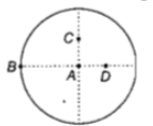A small object of uniform density rolls up a curved surface with an initial velocity v'. It reaches up to a maximum height of 3v2/4g with respect to the initial position. The object is

(a) ring

(b) solid sphere

(c) hollow sphere

(d) disc

Concept Questions :-

Moment of inertia
High Yielding Test Series + Question Bank - NEET 2020

Difficulty Level:

When a mass is rotating in a plane about a fixed point, its angular momentum is directed along

(a)a line perpendicular to the plane of rotation

(b)the line making an angle of $45°$ to the plane of rotation

(d)the tangent to the orbit

Concept Questions :-

Angular momentum
High Yielding Test Series + Question Bank - NEET 2020

Difficulty Level:

Two persons of mass 55 kg and 65 kg respectively, are at the opposite ends of a boat.The length of the boat is 3.0m and weighs 100 kg.The 55 kg man walks up to the 65 kg man and sits with him.If the boat is in still water the centre of mass of the system shifts by

(a)3.0m                            (b)2.3m

(c)zero                             (d)0.75m

Concept Questions :-

Center of mass
High Yielding Test Series + Question Bank - NEET 2020

Difficulty Level:

A solid cylinder of mass 3kg is rolling on a horizontal surface with velocity 4 $m{s}^{-1}.$ It collides with a horizontal spring of force constant 200 $N{m}^{-1}$. The maximum compression produced in the spring will be

1. 0.5 m

2. 0.6 m

3. 0.7 m

4. 0.2 m

Concept Questions :-

Rolling motion
High Yielding Test Series + Question Bank - NEET 2020

Difficulty Level:

Two spheres A and B of masses  respectively collide. A is at rest initially and B is moving with velocity v along x-axis. After collision B has a velocity $\frac{v}{2}$ in a direction perpendicular to the original direction.The mass A moves after collision in the direction

(a)same as that of B

(b)opposite to that of B

(c)

(d)

Concept Questions :-

Linear momentum
High Yielding Test Series + Question Bank - NEET 2020

Difficulty Level:

The moment of inertia of a uniform circular

disc is maximum about an axis perpendicular

to the disc and passing through(a) B                    (b) C

(c) D                    (d) A

Concept Questions :-

Moment of inertia
High Yielding Test Series + Question Bank - NEET 2020

Difficulty Level:

Three masses are placed on the x-axis:

300 g at origin, 500 g at x= 40 cm and 400g

at x=70 cm. The distance of the center of

mass from the origin is

(a) 40 cm                   (b) 45 cm

(c) 50 cm                   (d) 30 cm

Concept Questions :-

Center of mass
High Yielding Test Series + Question Bank - NEET 2020

Difficulty Level:

The instantaneous angular position of a point on

a rotating wheel is given by the equation

$\mathrm{\theta }\left(\mathrm{t}\right)=2{\mathrm{t}}^{3}-6{\mathrm{t}}^{2}$

The torque on the wheel becomes zero at

(a) t=0.5 s

(b) t=0.25 s

(c) t=2 s

(d) t=1 s

Concept Questions :-

Torque
High Yielding Test Series + Question Bank - NEET 2020

Difficulty Level:

The moment of inertia of a thin uniform rod of

mass M and length L about an axis passing

through its mid-point and perpendicular to its

length is ${\mathrm{I}}_{0}$. Its moment of inertia about an

axis passing through one of its ends and

perpendicular to its length is

(a) ${\mathrm{I}}_{0}+{\mathrm{ML}}^{2}/4$

(b) ${\mathrm{I}}_{0}+2{\mathrm{ML}}^{2}$

(c) ${\mathrm{I}}_{0}+{\mathrm{ML}}^{2}$

(d) ${\mathrm{I}}_{0}+{\mathrm{ML}}^{2}/2$

Concept Questions :-

Moment of inertia
High Yielding Test Series + Question Bank - NEET 2020

Difficulty Level:

A body of mass M hits normally a rigid wall with velocity v and bounces back with the same velocity. The impulse experienced by the body is

(a) 1.5 Mv                                                 (b) 2 Mv

(c) zero                                                     (d) Mv

Concept Questions :-

Linear momentum